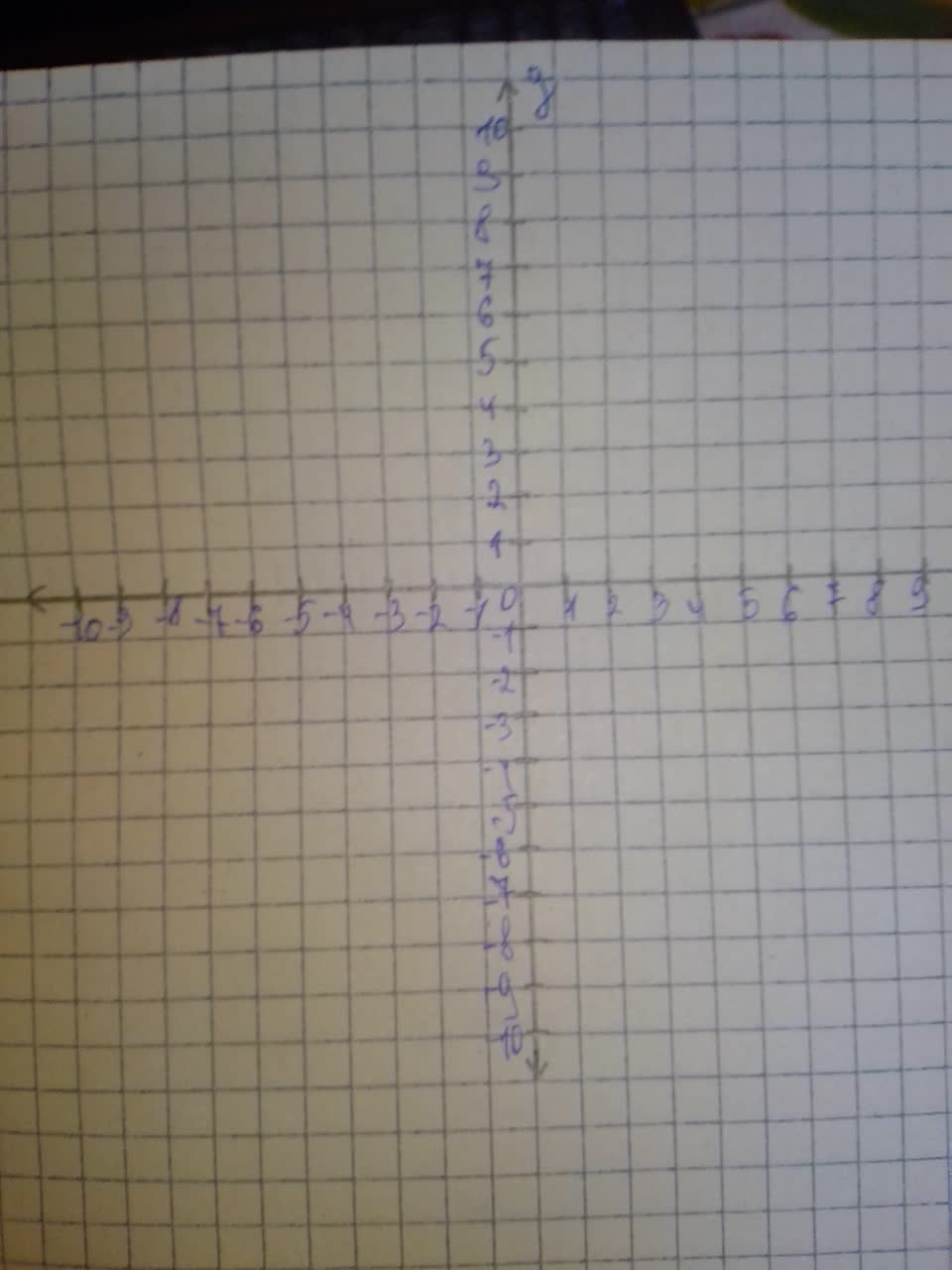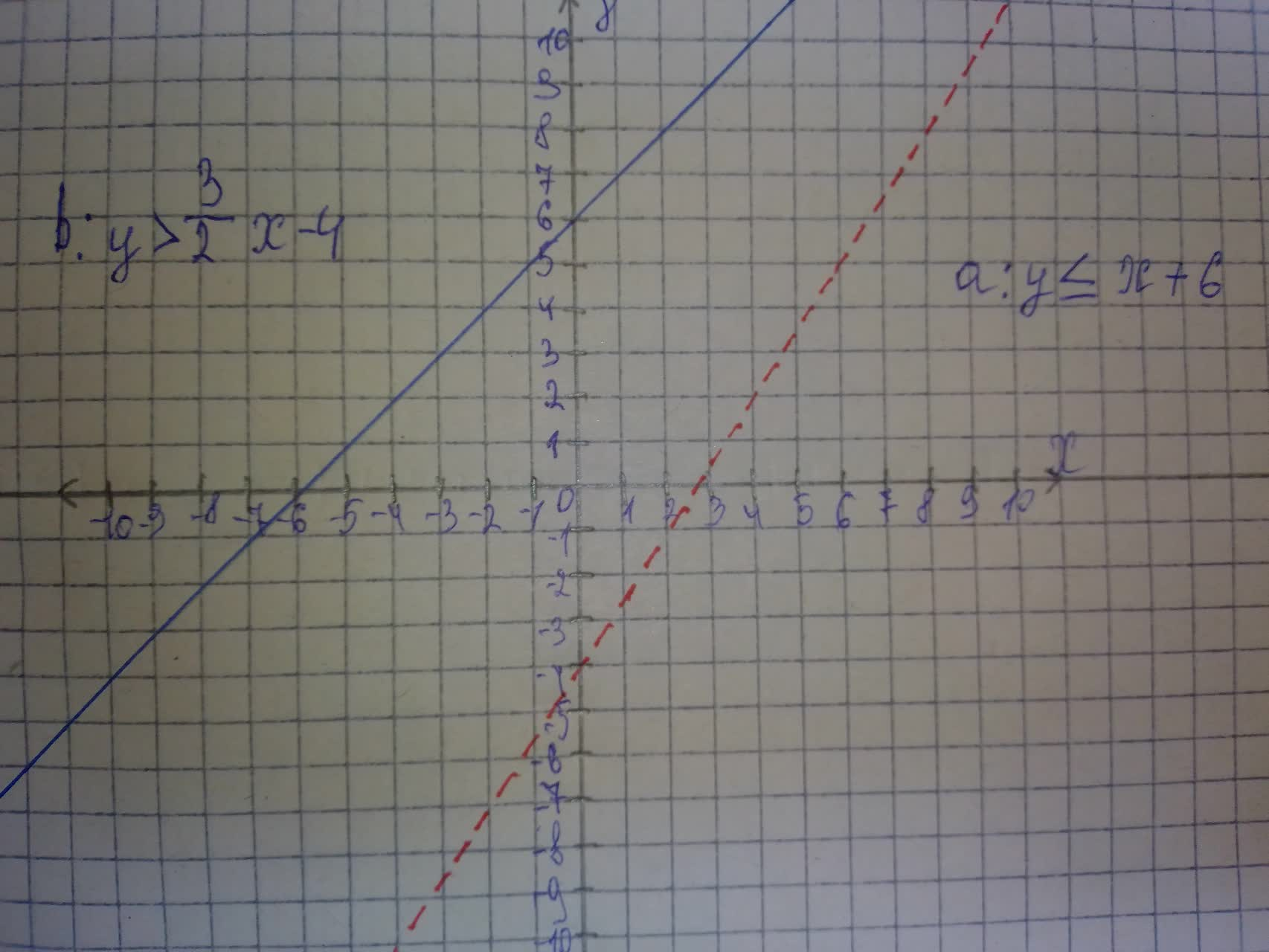Question# Solve the following systems of inequalities graphically on the set of axes below. y\leqx+6 y>-\frac{3}{2}x-4

Alternate coordinate systems
ANSWEREDSolve the following systems of inequalities graphically on the set of axes below.The state the following coordinates of a point in the solution say
$$\displaystyle{y}\leq{x}+{6}$$
$$\displaystyle{y}\succ{\frac{{{3}}}{{{2}}}}{x}-{4}$$The solutions are $$\displaystyle{\left({1},{0}\right)},{\left(-{2},{0}\right)},{\left({1},{1}\right)},{\left(-{3},{0}\right)},{\left({0},{0}\right)}$$